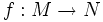# Critical point set is closed

(diff) ← Older revision | Latest revision (diff) | Newer revision → (diff)
Jump to: navigation, search

This article gives the statement and possibly proof of a theorem that discusses regular values, critical values, regular points or critical points of a smooth map between differential manifolds

## Statement

Let$f:M \to N$ be a smooth map of differential manifolds. Then, the set of critical points of$f$ is a closed subset of$M$; equivalently, the set of regular points of$f$ is an open subset of$M$.